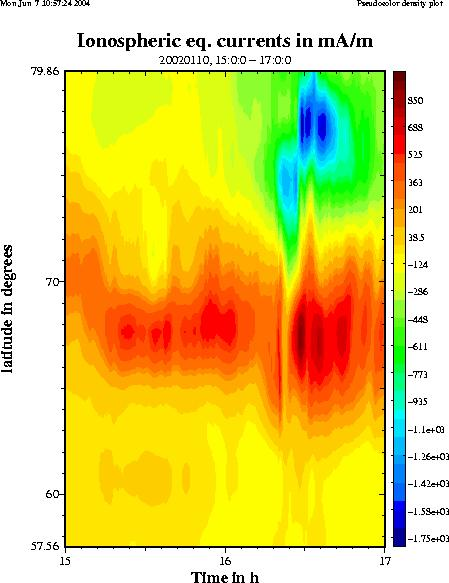# Calculate ionospheric equivalent currents (1D)

Please use the new versions:
1D equivalent currents
2D equivalent currents

From ground magnetometer data the ionospheric equivalent currents can be calculated. These are currents that flow only within the ionospheric plane (assumed at 100 km altitude) and cause the same magnetic field on the ground as the actual ionospheric current system (which may be three-dimensional). So please note: Ionospheric equivalent currents are not necessarily equal to the actual currents!

For the technique used here, we assume for simplicity that the currents are only varying in north-south direction, but not in east-west direction (electrojet-type situation). Only the east-west component of equivalent current is calculated.Example input data: X (northward) component data of IMAGE magnetometers on Jan 10th, 2002. Analysis interval: 15-17 UT. (Click to view a larger image). Example output plot: Ionospheric equivalent currents in east-west direction on Jan 10th 2002, 15-17 UT. (Click to view a larger version)

The results are displayed as colour plots that show the time evolution of the equivalent currents along a north-south profile. Red colours (positive numbers) mean eastward equivalent currents, blue colours (negative numbers) mean westward currents. Four plots are displayed:

• Ionospheric equivalent currents
• Ionospheric equivalent currents, only eastward component
• Ionospheric equivalent currents, only westward component
• Integrated ionospheric equivalent current, separately for eastward and westward components

To scale the magnetic data, you need to give a baseline period, i.e. magnetically quiet period close to the event you are going to analyse. To find one, use the IMAGE IL index plots.

More info may be found from 1D upward continuation page under "analysis methods".

### Event selection form

#### NOTE: The script cannot handle midnight crossings so the event start and event end days must be same.

Event period:
Year Month Day Hour Minute
Start time:
End time:
Baseline period:
Year Month Day Hour Minute
Start time:
End time:

Time resolution: seconds (must be integer multiple of 10)

(calculation will take a few seconds)

Updated: 28.7.2010 - LH